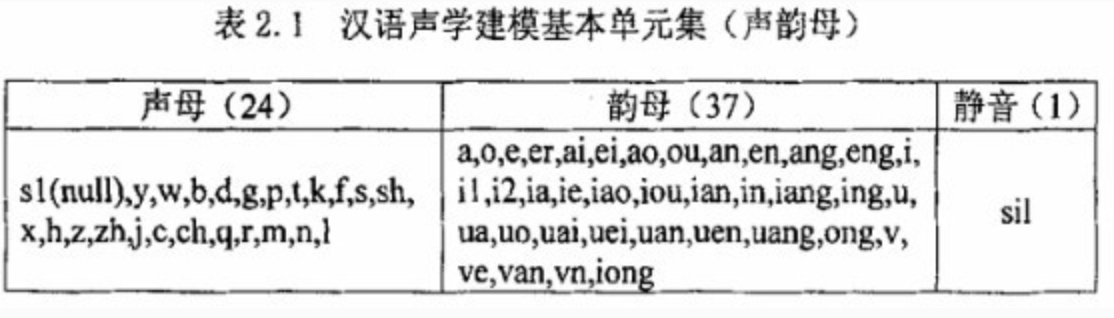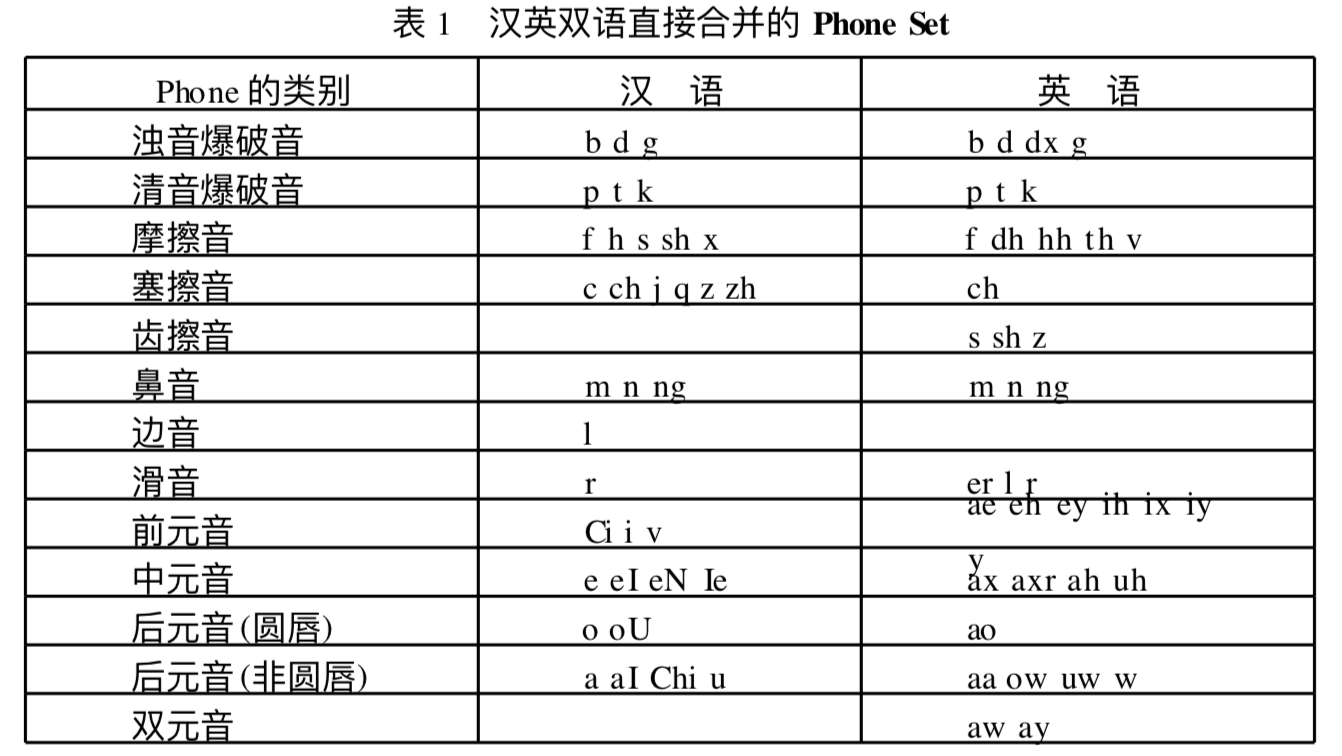2019-07-24 21:57:01 alice_tl 阅读数 176
• ###### C++语音识别开篇

本篇mark老师将教大家使用第三方库的调用来简单的实现语音识别。随着机器学习和人工智能的热闹，国内语音行业也可谓是百花齐放。 语音识别一个伟大的时代已在我们身边悄悄走来。

5905 人正在学习 去看看 杨波

### 发展阶段

50年代：贝尔实验室开始语音识别的研究，主要研究基于简单的孤立词的语音识别系统。实现针对特定说话人的十个英文数字的孤立词语音识别系统。

60年代：出现三个关键的技术，提出一种时间规整的机制，采用动态规划算法实现动态时间规整DTW技术，利用音素动态跟踪的方法进行连续语音识别的开创性工作。为语音识别的后来发展奠定了基础。

70年代：语音识别技术快速发展的时期。实现大规模语音识别，大词汇量，孤立词识别。

80年代：基于隐马尔科夫模型的声学建模和基于n．gram的语言模型。尝试大词汇量、非特定人连续语音识别。

90年代：语音识别技术基本成熟的时期，基于GMM-HMM的语音识别框架得到广泛使用和研究。

20年代初期：语音识别并没有得到非常好的应用，因为系统的错误率还很高。2006年开始再度兴起。

### 区别与联系

 类型 简称 目的 应用场景 语音识别 ASR 语音——》文字 生成字幕、智慧会场、语音唤醒、智能客服 声纹识别 VPR 识别说话人的身份 身份认证、公安刑侦 语音唤醒 KWS 语音——》指令 小度音箱、小爱音箱 语音合成 TTS 文字——》语音 智能音箱 语言处理 NLP 语音——》语言 同声翻译、自动阅卷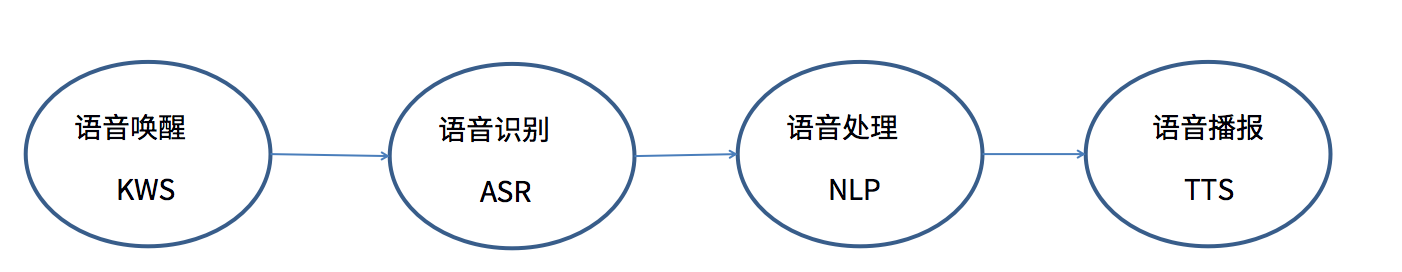阶段 唤醒KWS 语音识别ASR 语音处理NLP 语音播报TTS IN 关键字语音 用户指令语音 用户指令文字 播报文字 OUT 是否唤醒 文字 应对处理+播报文字 播报语音 示例 小爱同学 回复我在 现在几点了 Domin：time Intention：current time To_speek：现在时间是九点 播放MP3音频：现在时间是九点

### 语音识别的分类

 范围 概念 算法 应用场景 提供形式 封闭域识别 以预先指定的字/词集合为识别范围，对范围之外的语音会拒识 声学模型和语言模型进行裁剪，使得识别引擎的运算量变小 不涉及到多轮交互和多种语义说法的场景。 如电视盒子，智能音箱 一般将引擎封到嵌入式芯片或者本地化的SDK中，从而使识别过程完全脱离云端，摆脱对网络的依赖，并且不会影响识别率 开放域识别 无需预先指定识别词集合，在整个语言大集合范围中进行识别 声学模型和语音模型一般都比较大，引擎运算量也较大 实时性要求不高的客服语音质检，录制完的视频转字幕配置等。如会议字幕系统 基本上都只以云端形式提供（云端包括公有云形式和私有云形式），依赖于网络

 范围 概念 应用场景 孤立词识别 识别事先已知的孤立的词 如“开机”、“关机”等 关键词识别 检测针对的是连续语音，但它并不识别全部文字，而只是检测已知的若干关键词在何处出现 如在一段话中检测“计算机”、“世界”这两个词 连续语音识别 识别任意的连续语音， 如一个句子或一段话

2018-05-03 20:20:03 luodongri 阅读数 7028
• ###### C++语音识别开篇

本篇mark老师将教大家使用第三方库的调用来简单的实现语音识别。随着机器学习和人工智能的热闹，国内语音行业也可谓是百花齐放。 语音识别一个伟大的时代已在我们身边悄悄走来。

5905 人正在学习 去看看 杨波

# CTC算法概念

CTC算法全称叫：Connectionist temporal classification。从字面上理解它是用来解决时序类数据的分类问题。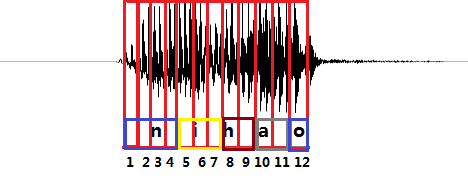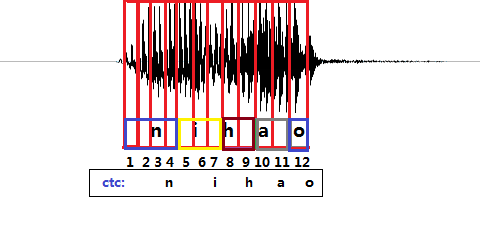CTC引入了blank（该帧没有预测值），每个预测的分类对应的一整段语音中的一个spike（尖峰），其他不是尖峰的位置认为是blank。对于一段语音，CTC最后的输出是spike（尖峰）的序列，并不关心每一个音素持续了多长时间。

# RNN+CTC模型的训练

$z=\left({z}_{1},{z}_{2},{z}_{3},...{z}_{U}\right)$$z=(z_1,z_2, z_3,...z_U)$表示这段样本语音对应的正确的音素。比如，一段发音“你好”的声音，经过MFCC计算后，得到特征$x$$x$， 它的文本信息是“你好”，对应的音素信息是$z=\left[n,i,h,a,o\right]$$z=[n,i,h,a,o]$(这里暂且将每个拼音的字母当做一个音素)。

$\sum _{t-1}^{T}{y}_{k}^{t}=1,{y}_{k}^{t}\ge 0$$\sum_{t-1}^{T}y^t_k=1, y^t_k\geq0$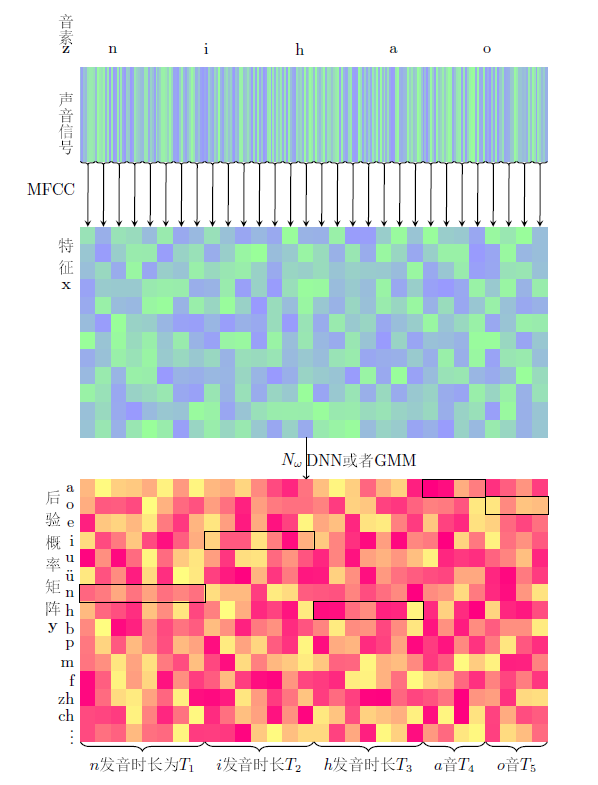## 路径π$\pi$$\pi$和B变换

$z\mathrm{\prime }=\left[\underset{{T}_{1}}{\underset{⏟}{n,n,n,...,n}},\underset{{T}_{2}}{\underset{⏟}{i,i,i,...i}},\underset{{T}_{3}}{\underset{⏟}{h,h,h,...h}},\underset{{T}_{4}}{\underset{⏟}{a,a,a,...,a}},\underset{{T}_{5}}{\underset{⏟}{o,o,o,...,o}}\right]$$z\prime = [\underbrace{n,n,n,...,n}_{T_1},\underbrace{i,i,i,...i}_{T_2},\underbrace{h,h,h,...h}_{T_3},\underbrace{a,a,a,...,a}_{T_4},\underbrace{o,o,o,...,o}_{T_5}]$

${T}_{1}+{T}_{2}+{T}_{3}+{T}_{4}+{T}_{5}=T$$T_1+T_2+T_3+T_4+T_5 = T$

$p\left(z\mathrm{\prime }|x\right)=p\left(z\mathrm{\prime }|y={N}_{w}\left(x\right)\right)={y}_{z{\mathrm{\prime }}_{1}}^{1}{y}_{z{\mathrm{\prime }}_{2}}^{2}{y}_{z{\mathrm{\prime }}_{3}}^{3}....{y}_{z{\mathrm{\prime }}_{T}}^{T}$$p(z\prime|x) = p(z\prime| y = N_w(x)) = y^1_{z\prime_1}y^2_{z\prime_2}y^3_{z\prime_3}....y^T_{z\prime_T}$ $\phantom{\rule{2em}{0ex}}\phantom{\rule{2em}{0ex}}\phantom{\rule{2em}{0ex}}\phantom{\rule{2em}{0ex}}\phantom{\rule{2em}{0ex}}\phantom{\rule{2em}{0ex}}\phantom{\rule{2em}{0ex}}\phantom{\rule{2em}{0ex}}\phantom{\rule{2em}{0ex}}\phantom{\rule{2em}{0ex}}$$\qquad\qquad\qquad\qquad\qquad\qquad\qquad\qquad\qquad\qquad$ (1)

$mi{n}_{w}-log\left({y}_{{z}_{1}^{\mathrm{\prime }}}^{1}.{y}_{{z}_{2}^{\mathrm{\prime }}}^{2}.{y}_{{z}_{3}^{\mathrm{\prime }}}^{3}...{y}_{{z}_{T}^{\mathrm{\prime }}}^{T}\right)$$min_w -log(y^1_{z^\prime_1}.y^2_{z^\prime_2}.y^3_{z^\prime_3}...y^T_{z^\prime_T})$ $\phantom{\rule{2em}{0ex}}\phantom{\rule{2em}{0ex}}\phantom{\rule{2em}{0ex}}\phantom{\rule{2em}{0ex}}\phantom{\rule{2em}{0ex}}\phantom{\rule{2em}{0ex}}\phantom{\rule{2em}{0ex}}\phantom{\rule{2em}{0ex}}\phantom{\rule{2em}{0ex}}\phantom{\rule{2em}{0ex}}$$\qquad\qquad\qquad\qquad\qquad\qquad\qquad\qquad\qquad\qquad$ (2)

subject to: $y={N}_{w}\left(x\right)$$y = N_w(x)$$\phantom{\rule{2em}{0ex}}\phantom{\rule{2em}{0ex}}\phantom{\rule{2em}{0ex}}\phantom{\rule{2em}{0ex}}\phantom{\rule{2em}{0ex}}\phantom{\rule{2em}{0ex}}\phantom{\rule{2em}{0ex}}\phantom{\rule{2em}{0ex}}\phantom{\rule{2em}{0ex}}\phantom{\rule{2em}{0ex}}$$\qquad\qquad\qquad\qquad\qquad\qquad\qquad\qquad\qquad\qquad$ (3)

$\frac{\mathrm{\partial }-log\left({y}_{{z}_{1}^{\mathrm{\prime }}}^{1}.{y}_{{z}_{2}^{\mathrm{\prime }}}^{2}.{y}_{{z}_{3}^{\mathrm{\prime }}}^{3}...{y}_{{z}_{T}^{\mathrm{\prime }}}^{T}\right)}{\mathrm{\partial }{y}_{k}^{t}}=$$\frac{\partial - log(y^1_{z^\prime_1}.y^2_{z^\prime_2}.y^3_{z^\prime_3}...y^T_{z^\prime_T})}{\partial y^t_k} =$$\left\{\begin{array}{l}-\frac{{y}_{z{\mathrm{\prime }}_{1}}^{1}...{y}_{z{\mathrm{\prime }}_{i-1}}^{i-1}.{y}_{z{\mathrm{\prime }}_{i+1}}^{i+1}....{y}_{z{\mathrm{\prime }}_{T}}^{T}}{{y}_{{z}_{1}^{\mathrm{\prime }}}^{1}.{y}_{{z}_{2}^{\mathrm{\prime }}}^{2}.{y}_{{z}_{3}^{\mathrm{\prime }}}^{3}...{y}_{{z}_{T}^{\mathrm{\prime }}}^{T}},\phantom{\rule{2em}{0ex}}if\phantom{\rule{2em}{0ex}}k=z{\mathrm{\prime }}_{i}\phantom{\rule{2em}{0ex}}and\phantom{\rule{2em}{0ex}}t=i\\ 0\phantom{\rule{2em}{0ex}}其他\end{array}$$\begin{cases} -\frac{y^1_{z\prime_1}...y^{i-1}_{z\prime_{i-1}}.y^{i+1}_{z\prime_{i+1}}....y^T_{z\prime_T}}{y^1_{z^\prime_1}.y^2_{z^\prime_2}.y^3_{z^\prime_3}...y^T_{z^\prime_T}} , \qquad if \qquad k = z\prime_i \qquad and \qquad t=i \\ 0 \qquad 其他\end{cases}$

$L=\left\{a,o,e,i,u,\stackrel{ˇ}{u},b,p,m,...\right\}$$L=\lbrace a, o, e, i, u, \check{u},b,p,m,...\rbrace$

$\pi =\left({\pi }_{1},{\pi }_{2},{\pi }_{3},...,{\pi }_{T}\right),{\pi }_{i}\in L$$\pi= (\pi_1, \pi_2, \pi_3, ..., \pi_T), \pi_i \in L$

${\pi }^{1}=\left(j,j,i,n,y,y,e,e,w,w,u,u,u,r,r,e,e,n,n,r,r,u,u,sh,sh,u,u,i,i\right)$$\pi^1= (j,j,i,n,y,y,e,e,w,w,u,u,u,r,r,e,e,n,n,r,r,u,u,sh,sh,u,u,i,i)$
${\pi }^{2}=\left(n,n,n,n,i,i,i,i,h,h,h,h,a,a,a,a,a,a,a,a,a,o,o,o,o,o,o,o,o,o\right)$$\pi^2= (n,n,n,n,i,i,i,i,h,h,h,h,a,a,a,a,a,a,a,a,a,o,o,o,o,o,o,o,o,o)$
${\pi }^{3}=\left(h,h,h,h,h,h,a,a,a,a,a,a,a,o,o,o,o,n,n,n,n,n,n,i,i,i,i,i,i,i\right)$$\pi^3= (h,h,h,h,h,h,a,a,a,a,a,a,a,o,o,o,o,n,n,n,n,n,n,i,i,i,i,i,i,i)$
${\pi }^{4}=\left(n,i,h,a,o,o,o,o,o,o,o,o,o,o,o,o,o,o,o,o,o,o,o,o,o,o,o,o,o,o,o\right)$$\pi^4= (n,i,h,a,o,o,o,o,o,o,o,o,o,o,o,o,o,o,o,o,o,o,o,o,o,o,o,o,o,o,o)$
${\pi }^{5}=\left(n,n,n,n,n,n,n,n,n,n,n,n,n,n,n,n,n,n,n,n,n,n,n,n,i,h,a,o\right)$$\pi^5= (n,n,n,n,n,n,n,n,n,n,n,n,n,n,n,n,n,n,n,n,n,n,n,n,i,h,a,o)$
${\pi }^{6}=\left(n,n,n,i,i,i,h,h,h,h,h,a,,o,o,o,o,o,o,o,o,o,o,o,o,o,o,o,o,o,o\right)$$\pi^6= (n,n,n,i,i,i,h,h,h,h,h,a,,o,o,o,o,o,o,o,o,o,o,o,o,o,o,o,o,o,o)$

$B\left(a,a,a,b,b,b,c,c,d\right)=\left(a,b,c,d\right)$$B(a,a,a,b,b,b,c,c,d) = (a,b,c,d)$

$B\left({\pi }^{1}\right)=\left(j,i,n,y,e,w,u,r,e,n,r,u,s,h,u,i\right)$$B(\pi^1) = (j,i,n,y,e,w,u,r,e,n,r,u,s,h,u,i)$
$B\left({\pi }^{2}\right)=\left(n,i,h,a,\right)$$B(\pi^2) = (n,i,h,a,)$
$B\left({\pi }^{3}\right)=\left(h,a,o,n,i\right)$$B(\pi^3) = (h,a,o,n,i)$
$B\left({\pi }^{4}\right)=\left(n,i,h,a,o\right)$$B(\pi^4) = (n,i,h,a,o)$
$B\left({\pi }^{5}\right)=\left(n,i,h,a,o\right)$$B(\pi^5) = (n,i,h,a,o)$
$B\left({\pi }^{6}\right)=\left(n,i,h,a,o\right)$$B(\pi^6) = (n,i,h,a,o)$

$p\left(\pi |x\right)=p\left(\pi |y={N}_{w}\left(x\right)\right)=p\left(\pi |y\right)=\prod _{t=1}^{T}{y}_{{\pi }_{t}}^{t}$$p(\pi|x) = p(\pi| y = N_w(x)) = p(\pi| y)=\prod_{t=1}^{T} y^t_{\pi_t}$

$ma{x}_{w}p\left(z|y={N}_{w}\left(x\right)\right)=p\left(z|x\right)=\sum _{B\left(\pi \right)=z}p\left(\pi |x\right)$$max_w p(z|y=N_w(x)) = p(z|x)= \sum_{B(\pi)=z}p(\pi|x)$ $\phantom{\rule{2em}{0ex}}\phantom{\rule{2em}{0ex}}\phantom{\rule{2em}{0ex}}$$\qquad\qquad\qquad$ (4)

## 训练实施方法

CTC的训练过程是通过$\frac{\mathrm{\partial }p\left(z|x\right)}{\mathrm{\partial }w}$$\frac {\partial p(z|x)}{\partial w}$调整w的值使得4中的目标值最大，而计算的过程如下：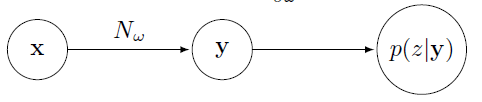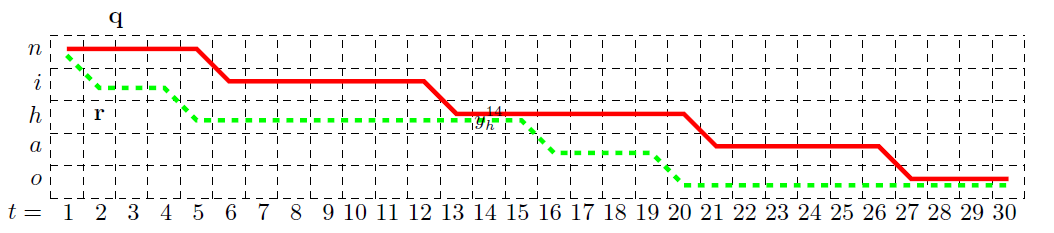$\frac{\mathrm{\partial }p\left(z|y\right)}{\mathrm{\partial }{y}_{h}^{14}}$$\frac {\partial p(z|y)}{\partial y^{14}_h}$

= $\frac{\mathrm{\partial }\sum _{B\left(\pi \right)=z}p\left(\pi |y\right)}{\mathrm{\partial }{y}_{h}^{14}}$$\frac {\partial \sum_{B(\pi)=z}p(\pi|y)}{\partial y^{14}_h}$

= $\frac{\mathrm{\partial }\sum _{B\left(\pi \right)=z}\prod _{t=1}^{T}{y}_{{\pi }_{t}}^{t}}{\mathrm{\partial }{y}_{h}^{14}}$$\frac {\partial \sum_{B(\pi)=z}\prod_{t=1}^T y^t_{\pi_t}}{\partial y^{14}_h}$
=$\frac{\stackrel{和{y}_{h}^{14}有关的项}{\stackrel{⏞}{\mathrm{\partial }\sum _{B\left(\pi \right)=z,{\pi }_{14}=h}\prod _{t=1}^{T}{y}_{{\pi }_{t}}^{t}}}+\stackrel{和{y}_{h}^{14}无关的项}{\stackrel{⏞}{\mathrm{\partial }\sum _{B\left(\pi \right)=z,{\pi }_{14}\ne h}\prod _{t=1}^{T}{y}_{{\pi }_{t}}^{t}}}}{\mathrm{\partial }{y}_{h}^{14}}$$\frac {\overbrace{\partial \sum_{B(\pi)=z,\pi_{14}=h}\prod_{t=1}^T y^t_{\pi_t}}^{和y^{14}_h有关的项} + \overbrace{\partial \sum_{B(\pi)=z,\pi_{14} \neq h}\prod_{t=1}^T y^t_{\pi_t}}^{和y^{14}_h无关的项}}{\partial y^{14}_h}$

=$\frac{\mathrm{\partial }\sum _{B\left(\pi \right)=z,{\pi }_{14}=h}\prod _{t=1}^{T}{y}_{{\pi }_{t}}^{t}}{\mathrm{\partial }{y}_{h}^{14}}$$\frac {\partial \sum_{B(\pi)=z,\pi_{14}=h}\prod_{t=1}^T y^t_{\pi_t}}{\partial y^{14}_h}$

$\underset{路径q的概率}{\underset{⏟}{{y}_{q1}^{1}..{y}_{q13}^{13}.{y}_{h}^{14}.{y}_{q15}^{15}....{y}_{q30}^{30}}}$$\underbrace{y^1_{q1}..y^{13}_{q13}.y^{14}_{h}.y^{15}_{q15}....y^{30}_{q30}}_{路径q的概率}$

+$\underset{路径{q}_{1:14}+{r}_{14:30}的概率}{\underset{⏟}{{y}_{q1}^{1}..{y}_{q13}^{13}.{y}_{h}^{14}.{y}_{r15}^{15}....{y}_{r30}^{30}}}$$\underbrace{y^1_{q1}..y^{13}_{q13}.y^{14}_{h}.y^{15}_{r15}....y^{30}_{r30}}_{路径q_{1:14}+r_{14:30}的概率}$

+$\underset{路径{r}_{1:14}+{q}_{14:30}的概率}{\underset{⏟}{{y}_{r1}^{1}..{y}_{r13}^{13}.{y}_{h}^{14}.{y}_{q15}^{15}....{y}_{q30}^{30}}}$$\underbrace{y^1_{r1}..y^{13}_{r13}.y^{14}_{h}.y^{15}_{q15}....y^{30}_{q30}}_{路径r_{1:14}+q_{14:30}的概率}$

+$\underset{路径r的概率}{\underset{⏟}{{y}_{r1}^{1}..{y}_{r13}^{13}.{y}_{h}^{14}.{y}_{r15}^{15}....{y}_{r30}^{30}}}$$\underbrace{y^1_{r1}..y^{13}_{r13}.y^{14}_{h}.y^{15}_{r15}....y^{30}_{r30}}_{路径r的概率}$

=$\left({y}_{q1}^{1}....{y}_{q13}^{13}+{y}_{r1}^{1}.....{y}_{r13}^{13}\right).{y}_{h}^{14}.\left({y}_{q15}^{15}....{y}_{q15}^{30}+{y}_{r15}^{15}....{y}_{r30}^{30}\right)$$(y^1_{q1}....y^{13}_{q13} + y^1_{r1}.....y^{13}_{r13}).y^{14}_h.(y^{15}_{q15}....y^{30}_{q15}+y^{15}_{r15}....y^{30}_{r30})$

$\sum _{B\left(\pi \right)=z,{\pi }_{14}=h}\prod _{t=1}^{T}{y}_{{\pi }_{t}}^{t}=\left(前置项\right).{y}_{h}^{14}.$$\sum_{B(\pi)=z,\pi_{14}=h}\prod_{t=1}^T y^t_{\pi_t}= (前置项).y^{14}_h.$(后置项)$定义： ${\alpha }_{\left(}14\right)\left(h\right)=\left(前置项\right).{y}_{h}^{14}=\sum _{B\left({\pi }_{1:14}\right)=\left[n,i,h\right]}\prod _{t\mathrm{\prime }=1}^{t}{y}_{{\pi }_{t\mathrm{\prime }}}^{t\mathrm{\prime }}$$\alpha_(14)(h)=(前置项).y^{14}_h = \sum_{B(\pi_{1:14})=[n,i,h] }\prod_{t\prime=1}^t y^{t\prime}_{\pi_{t\prime}}$ 该值可以理解为从初始到${y}_{h}^{14}$$y^{14}_h$这一段里，所有正向路径的概率之和。并且发现，${\alpha }_{14}\left(h\right)$$\alpha_{14}(h)$可以由${\alpha }_{13}\left(h\right)$$\alpha_{13}(h)$${\alpha }_{13}\left(i\right)$$\alpha_{13}(i)$递推得到，即： ${\alpha }_{14}\left(h\right)=\left({\alpha }_{13}\left(h\right)+{\alpha }_{13}\left(i\right)\right){y}_{h}^{14}$$\alpha_{14}(h) = (\alpha_{13}(h) + \alpha_{13}(i))y^{14}_h$ 该递推公式的含义是，只是在$t=13$$t=13$时发音是“h”或“i”，在$t=14$$t=14$时才有可能发音是“h”。那么在$t=14$$t=14$时刻发音是“h”的所有正向路径概率${\alpha }_{14}\left(h\right)$$\alpha_{14}(h)$就等于在$t=13$$t=13$时刻，发音为“h”的正向概率${\alpha }_{13}\left(h\right)$$\alpha_{13}(h)$加上发音为“i”的正向概率${\alpha }_{13}\left(i\right)$$\alpha_{13}(i)$，再乘以当前音素被判断为“h”的概率${y}_{h}^{14}$$y^{14}_h$。由此可知，每个${\alpha }_{t}\left(s\right)$$\alpha_t(s)$都可以由${\alpha }_{t-1}\left(s\right)$$\alpha_{t-1}(s)$${\alpha }_{t-1}\left(s-1\right)$$\alpha_{t-1}(s-1)$两个值得到。$\alpha$$\alpha$的递推流程如下图所示：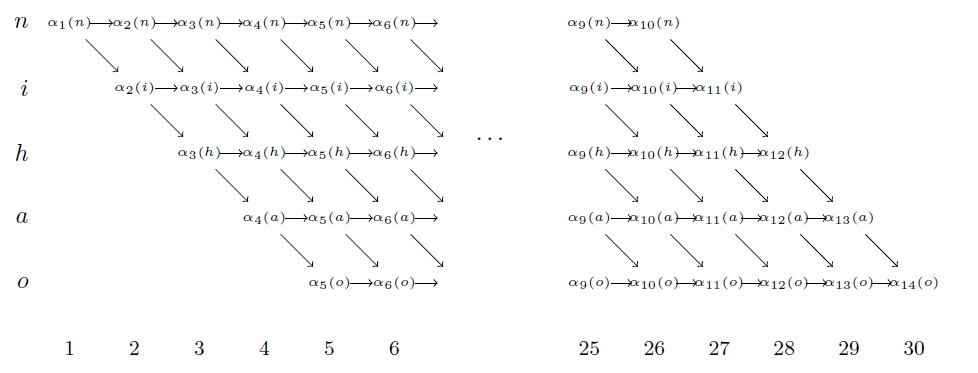即每个值都由上一个时刻的一个或者两个值得到，总计算量大约为$2.T.音素个数$$2.T.音素个数$。类似的，定义${\beta }_{t}\left(s\right)$$\beta_t(s)$， 递推公式为： ${\beta }_{14}\left(h\right)=\left({\beta }_{15}\left(h\right)+{\beta }_{15}\left(a\right)\right){y}_{h}^{14}$$\beta_{14}(h)=(\beta_{15}(h) + \beta_{15}(a))y^{14}_h$ 因此有： $\sum _{B\left(\pi \right)=z,{\pi }_{14}=h}\prod _{t=1}^{T}{y}_{{\pi }_{t}}^{t}=\left(前置项\right).{y}_{h}^{14}.$$\sum_{B(\pi)=z,\pi_{14}=h}\prod_{t=1}^T y^t_{\pi_t}= (前置项).y^{14}_h.$(后置项)$

=$\frac{{\alpha }_{14}\left(h\right)}{{y}_{h}^{14}}.{y}_{h}^{14}.\frac{{\beta }_{14}\left(h\right)}{{y}_{h}^{14}}$$\frac{\alpha_{14}(h)}{y^{14}_h}. y^{14}_h.\frac{\beta_{14}(h)}{y^{14}_h}$

=$\frac{{\alpha }_{14}\left(h\right){\beta }_{14}\left(h\right)}{{y}_{h}^{14}}$$\frac{\alpha_{14}(h)\beta_{14}(h)}{y^{14}_h}$

$\frac{\mathrm{\partial }p\left(z|y\right)}{\mathrm{\partial }{y}_{h}^{14}}$$\frac {\partial p(z|y)}{\partial y^{14}_h}$

= $\frac{\sum _{B\left(\pi \right)=z,{\pi }_{14}=h}\prod _{t=1}^{T}{y}_{{\pi }_{t}}^{t}}{\mathrm{\partial }{y}_{h}^{14}}$$\frac {\sum_{B(\pi)=z,\pi_{14}=h}\prod_{t=1}^T y^t_{\pi_t}}{\partial y^{14}_h}$

= $\frac{\frac{{\alpha }_{14}\left(h\right)}{{y}_{h}^{14}}.{y}_{h}^{14}.\frac{{\beta }_{14}\left(h\right)}{{y}_{h}^{14}}}{\mathrm{\partial }{y}_{h}^{14}}$$\frac {\frac{\alpha_{14}(h)}{y^{14}_h}. y^{14}_h.\frac{\beta_{14}(h)}{y^{14}_h}}{\partial y^{14}_h}$

=$\frac{{\alpha }_{14}\left(h\right){\beta }_{14}\left(h\right)}{{\left({y}_{h}^{14}\right)}^{2}}$$\frac{\alpha_{14}(h)\beta_{14}(h)}{{(y^{14}_h)}^2}$

2019-08-14 11:57:52 asrgreek 阅读数 926
• ###### C++语音识别开篇

本篇mark老师将教大家使用第三方库的调用来简单的实现语音识别。随着机器学习和人工智能的热闹，国内语音行业也可谓是百花齐放。 语音识别一个伟大的时代已在我们身边悄悄走来。

5905 人正在学习 去看看 杨波

• 模型参数变多，训练慢
• 梯度信息回流受阻，导致梯度消失

#### 一、LSTMP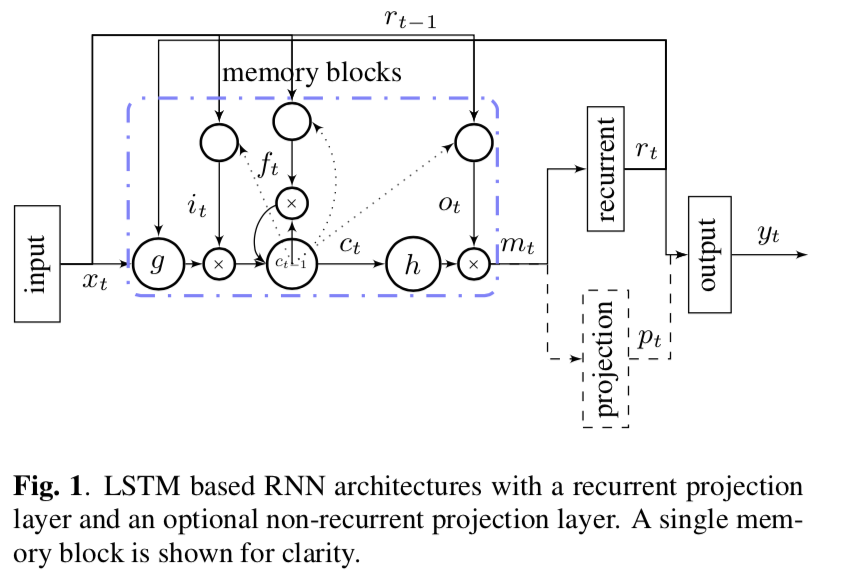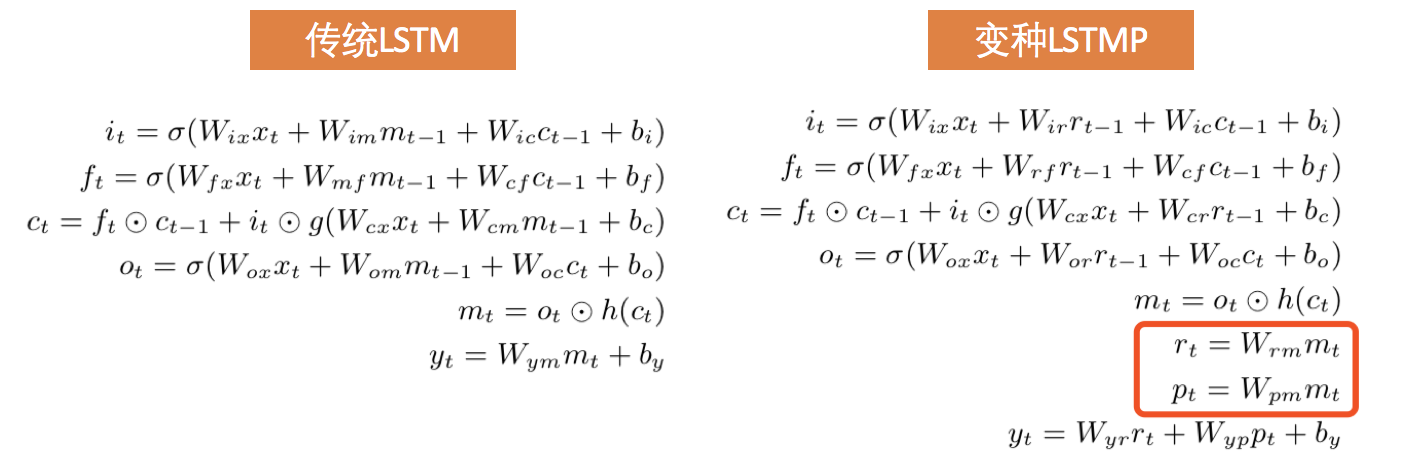1、$r_{t}$表示的是recurrent projection，也就是说$r_{t}$会作为下一个时间点各个门的输入；
2、$p_{t}$表示的是non-recurrent projection，这个映射可有可无，对结果影响不大。

1、《LONG SHORT-TERM MEMORY BASED RECURRENT NEURAL NETWORK ARCHITECTURES FOR LARGE VOCABULARY SPEECH RECOGNITION》
2、《Long Short-Term Memory Recurrent Neural Network Architectures for Large Scale Acoustic Modeling》

#### 二、Highway LSTM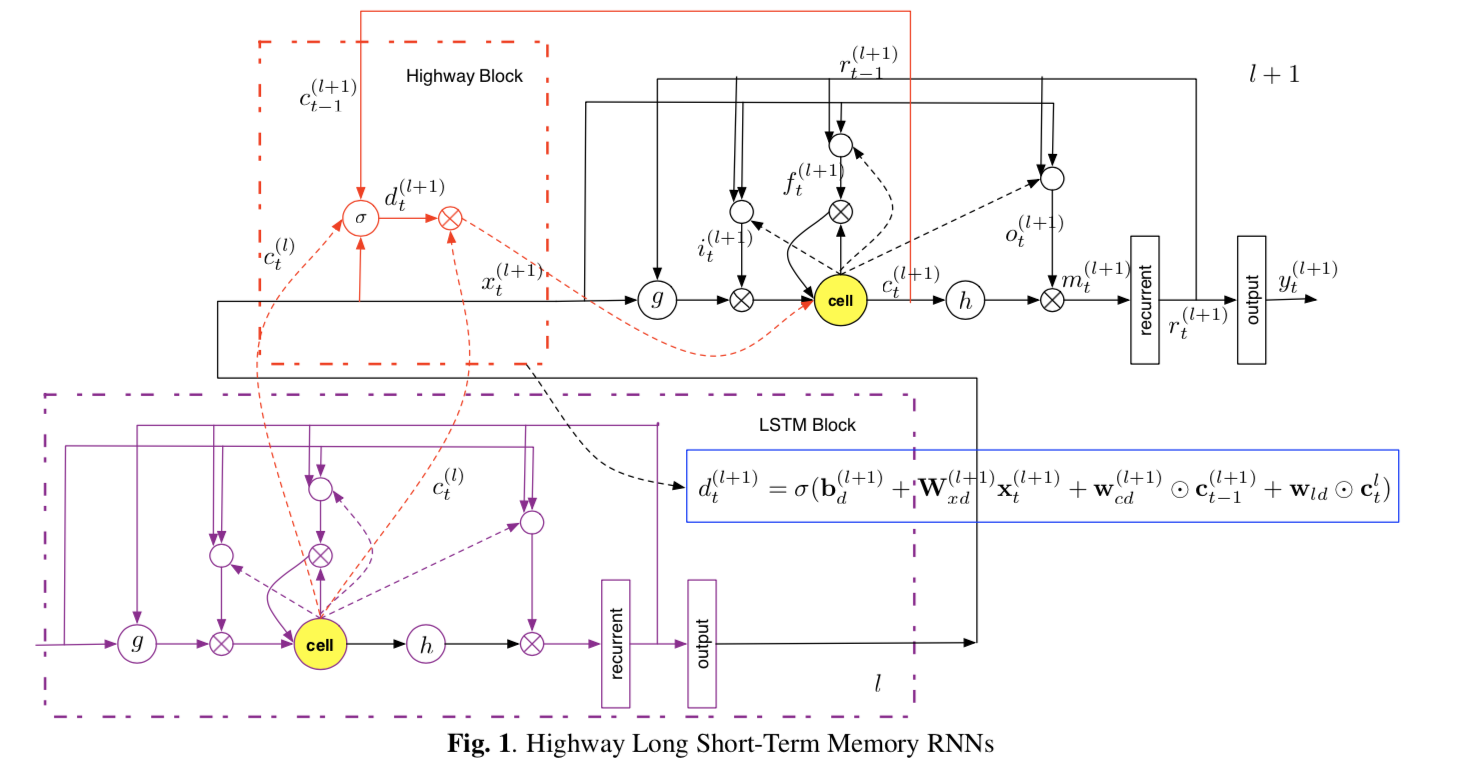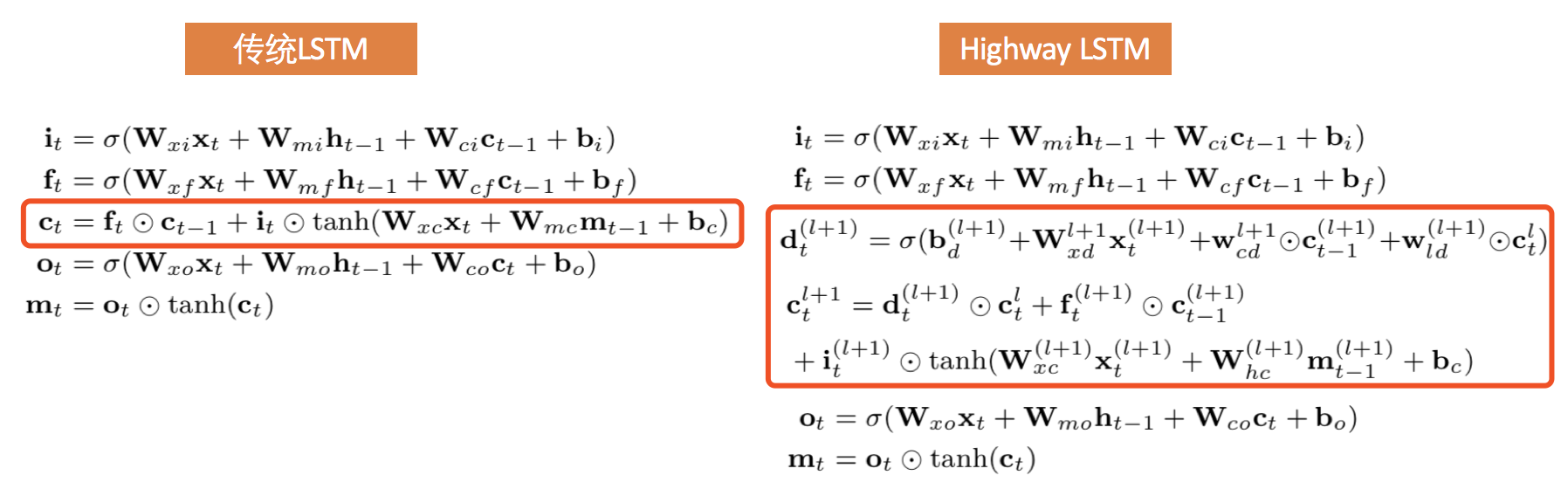1、《Highway long short-term memory RNNs for distant speech recognition》

#### 三、Residual LSTM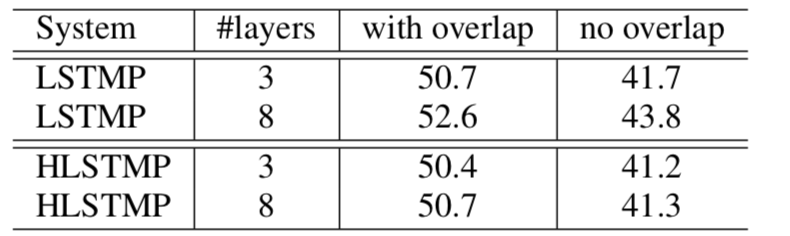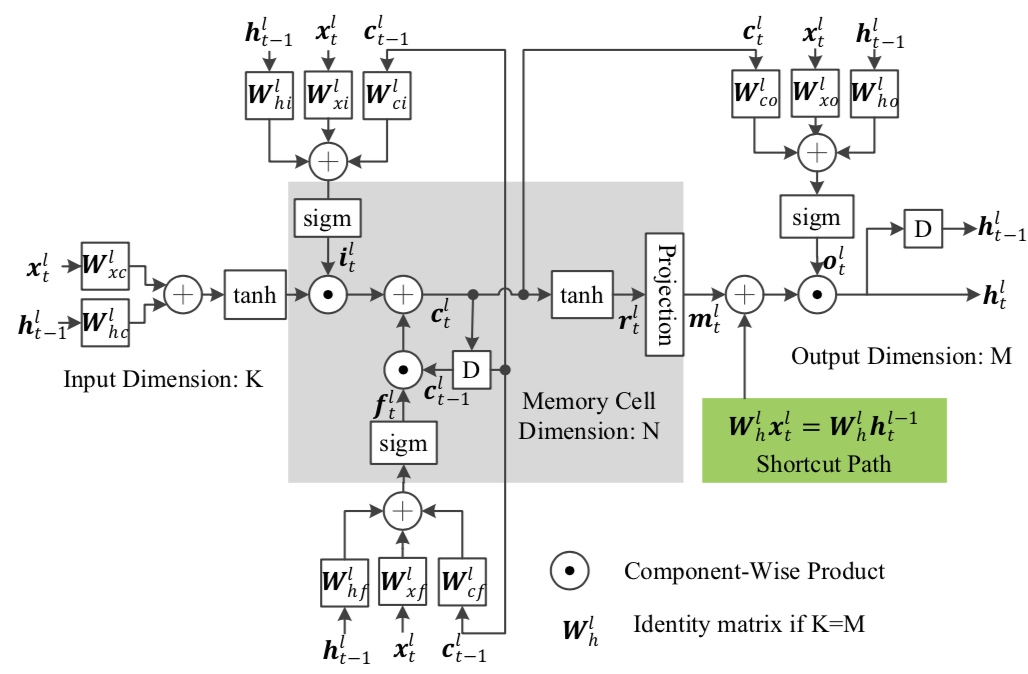1、Residual LSTM有一条空间上的捷径(对应绿色方块的Shortcut Path)。绿色方块怎么理解呢？它表达的是l层的输入$x_{t}^{l}$是来自l-1层的输出$h_{t}^{l-1}$。这里的空间指的是不同层的LSTM之间(论文中空间上的捷径用a spatial shortcut表达)。当然Residual LSTM也有一条时间上的捷径，怎么体现呢？图中l层t时刻的细胞状态依赖于l层t-1时刻的细胞状态，因此说它也提供了一条时间上的捷径(这一点传统LSTM也有，论文中时间上的捷径用a temporal shortcut path表示)
2、与Highway LSTM不同，Highway LSTM通过额外引入了carry gate来限制空间上的捷径的畅通程度。而Residual LSTM则直接使用output gate代替carry gate来限制空间上的捷径的畅通程度。通过这个代替，能有效超过减少10%的参数数量。
3、与Highway LSTM不同，Highway LSTM的时间捷径和空间捷径是应用在同一个对象上，即细胞状态。而Residual LSTM的时间捷径是应用在细胞状态，而空间捷径是应用在输出层。

Compared with the previous work, high- way LSTM, residual LSTM separates a spatial shortcut path with temporal one by using output layers, which can help to avoid a conflict between spatial and temporal-domain gradient flows.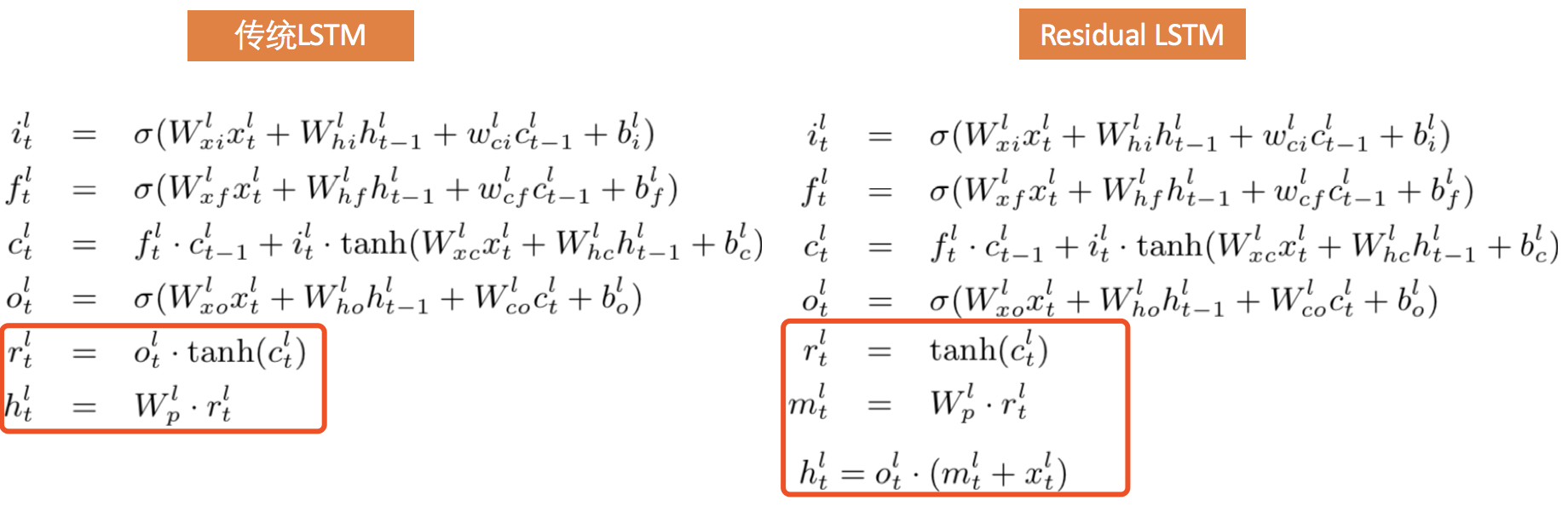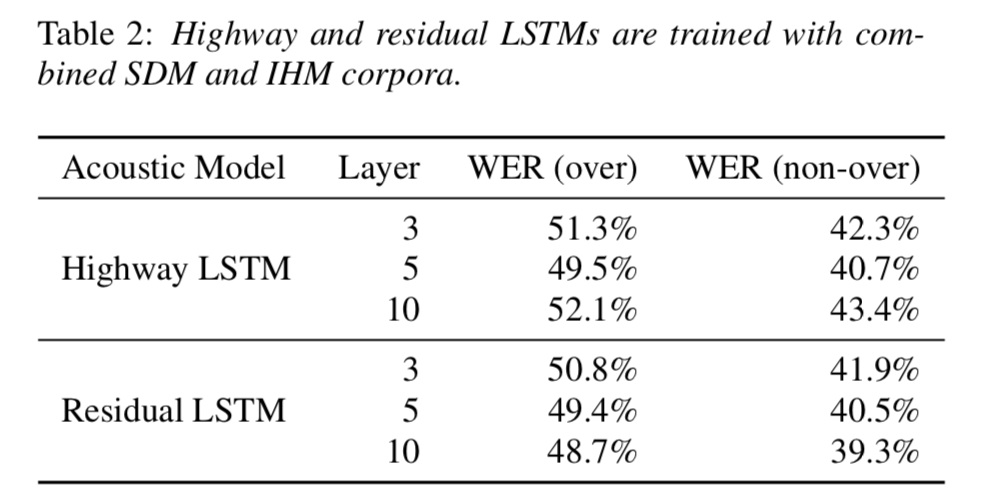1、《Design of a Deep Recurrent Architecture for Distant Speech Recognition》

2018-01-05 10:58:01 w_manhong 阅读数 13050
• ###### C++语音识别开篇

本篇mark老师将教大家使用第三方库的调用来简单的实现语音识别。随着机器学习和人工智能的热闹，国内语音行业也可谓是百花齐放。 语音识别一个伟大的时代已在我们身边悄悄走来。

5905 人正在学习 去看看 杨波

1. 能将语音信号转换为计算机能够处理的语音特征向量

2. 能够符合或类似人耳的听觉感知特性

3. 在一定程度上能够增强语音信号、抑制非语音信号

（1）线性预测分析（LinearPredictionCoefficients，LPC）

拟人类的发声原理，通过分析声道短管级联的模型得到的。假设系统的传递函数跟全极点的数字滤波器是相似的，通常用12-16个极点就可以描述语音信号的特征。所以对于n时刻的语音信号，我们可以用之前时刻的信号的线性组合近似的模拟。然后计算语音信号的采样值和线性预测的采样值。并让这两者之间达到均方的误差（MSE）最小，就可以得到LPC。

（2）感知线性预测系数（PerceptualLinearPredictive，PLP）

一种基于听觉模型的特征参数。该参数是一种等效于LPC的特征，也是全极点模型预测多项式的一组系数。不同之处是PLP是基于人耳听觉，通过计算应用到频谱分析中，将输入语音信号经过人耳听觉模型处理，替代LPC所用的时域信号，这样的优点是有利于抗噪语音特征的提取。

（3）Tandem特征和Bottleneck特征

这是两种利用神经网络提取的两类特征。Tandem特征是神经网络输出层节点对应类别的后验概率向量降维并与MFCC或者PLP等特征拼接得到。Bottleneck特征是用一种特殊结构的神经网络提取，这种神经网络的其中一个隐含层节点数目比其他隐含层小的多，所以被称之为Bottleneck（瓶颈）层，输出的特征就是Bottleneck特征。

（4）基于滤波器组的Fbank特征（Filterbank）

亦称MFSC，Fbank特征的提取方法就是相当于MFCC去掉最后一步的离散余弦变换，跟MFCC特征相比，Fbank特征保留了更多的原始语音数据。

（5）线性预测倒谱系数（LinearPredictiveCepstralCoefficient，LPCC）

基于声道模型的重要特征参数。LPCC是丢弃了信号生成过程中的激励信息。之后用十多个倒谱系数可以代表共振峰的特性。所以可以在语音识别中取得很好的性能。

（6）梅尔频率倒谱系数（MelFrequencyCepstrumCoefficient，MFCC）

基于人耳听觉特性，梅尔频率倒谱频带划分是在Mel刻度上等距划分的，频率的尺度值与实际频率的对数分布关系更符合人耳的听觉特性，所以可以使得语音信号有着更好的表示。1980年由Davis和Mermelstein搞出来的。从那时起。在语音识别领域，MFCC可谓是鹤立鸡群，一枝独秀。

Q: MFCC为何一枝独秀

人通过声道产生声音，声道的shape决定了发出怎样的声音。声道的shape包括舌头，牙齿等。如果我们可以准确的知道这个形状，那么我们就可以对产生的音素phoneme进行准确的描述。声道的形状在语音短时功率谱的包络中显示出来。而MFCC就是一种准确描述这个包络的一种特征。

处理语音信号，如何去描述它很重要，因为不同的描述方式放映它不同的信息,而声谱图的描述方式是最利于观测和理解的。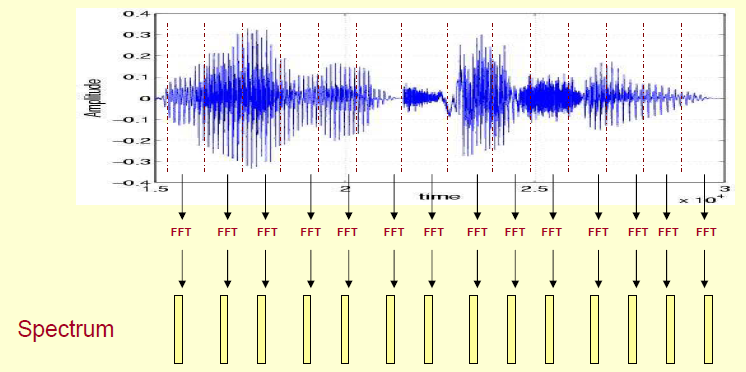由上图可知，这段语音被分为很多帧，每帧语音都对应于一个频谱（通过短时FFT计算），频谱表示频率与能量的关系。在实际使用中，频谱图有三种，即线性振幅谱、对数振幅谱、自功率谱（对数振幅谱中各谱线的振幅都作了对数计算，所以其纵坐标的单位是dB（分贝）。这个变换的目的是使那些振幅较低的成分相对高振幅成分得以拉高，以便观察掩盖在低幅噪声中的周期信号）。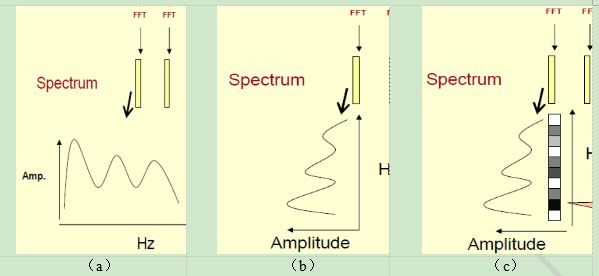先将其中一帧语音的频谱通过坐标表示出来，如上图（a）。旋转90度，得到图（b）。把这些幅度映射到一个灰度级表示，得到了图（c）。这样操作的原因是为了增加时间维度，，得到一个随着时间变化的频谱图，这个就是描述语音信号的声谱图（spectrogram）。这样就可以显示一段语音而不是一帧语音的频谱，而且可以直观的看到静态和动态的信息。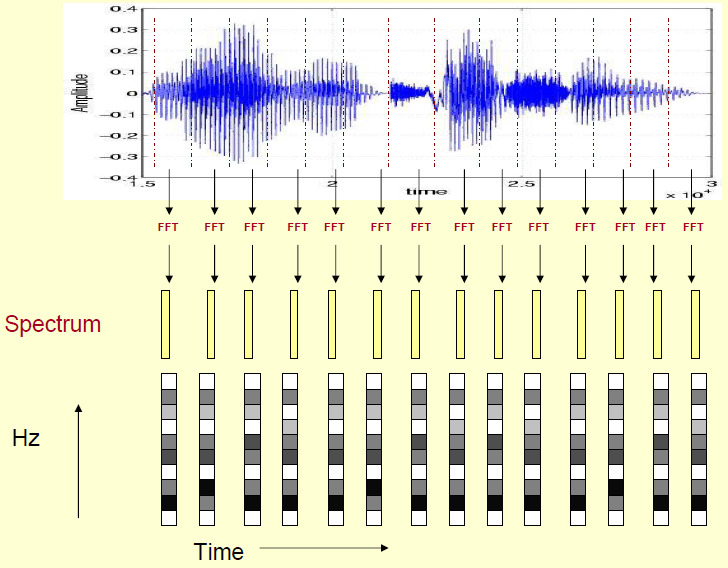下面是一个语音的频谱图。峰值就表示语音的主要频率成分，我们把这些峰值称为共振峰（formants），而共振峰就是携带了声音的辨识属性，用它就可以识别不同的声音。因此，需要把它提取出来。要提取的不仅是共振峰的位置，还得提取它们转变的过程。所以我们提取的是频谱的包络（SpectralEnvelope）。这包络就是一条连接这些共振峰点的平滑曲线。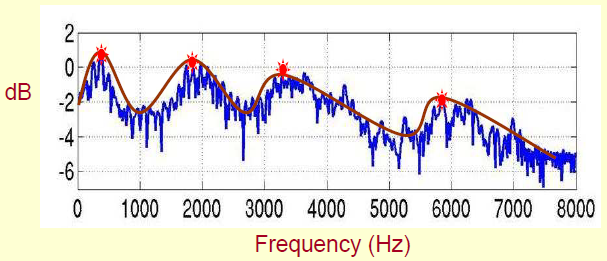由上图可以看出，原始的频谱由两部分组成：包络和频谱的细节。因此需要把这两部分分离开，就可以得到包络了。按照下图的方式进行分解，在给定logX[k]的基础上，求得logH[k]和logE[k]满足logX[k]=logH[k]+logE[k]。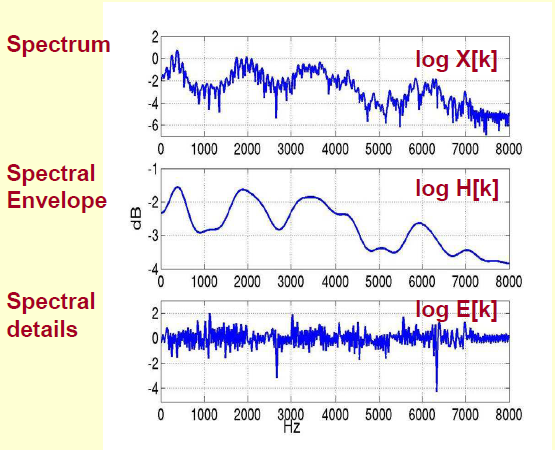由上面这个图我们可以看到，包络主要是低频成分，而高频主要是频谱的细节。把它俩叠加起来就是原来的频谱信号了。即，h[k]是x[k]的低频部分，因此将x[k]通过一个低通滤波器就可以得到h[k]了，也就是频谱的包络。

以上解卷过程的专业术语叫做同态信号处理，（另一种方法为基于线性变换）。语音本身可以看成是声道冲击信息（包括话者个性信息、语义信息，表现为频谱低频成分）经过声门激励的一个响应函数，在时域上表现为卷积形式。为将二者分离开来，求得声道共振特征和基音周期，需要把这种非线性问题转化为线性问题第一步通过FFT将其变成了乘性信号（时域的卷积相当于频域的乘积）；第二步通过取对数将乘性信号转化为加性信号；第三步进行逆变换，使其恢复为卷性信号。此时，虽然前后均是时域序列，但它们所处的离散时域显然不同，所以后者称为倒谱频域。计算过程如下图所示。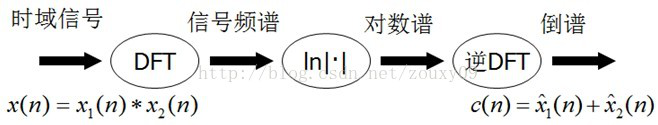2019-11-11 19:42:43 qq_40168949 阅读数 34
• ###### C++语音识别开篇

本篇mark老师将教大家使用第三方库的调用来简单的实现语音识别。随着机器学习和人工智能的热闹，国内语音行业也可谓是百花齐放。 语音识别一个伟大的时代已在我们身边悄悄走来。

5905 人正在学习 去看看 杨波

#### 声学模型

##### 英文的建模单元

kaldi工具箱timit例子中找到的现在英语标准的61-48-39的建模单元，从61个phone的基础上把发音相似的phone归成一个phone，降建模单元减为48个，同样的思路，再减为39个。要用这个建模单元表时，你得首先保证你数据库的标注文本都是用这样的phone标的。【2】

##### 中文的建模单元